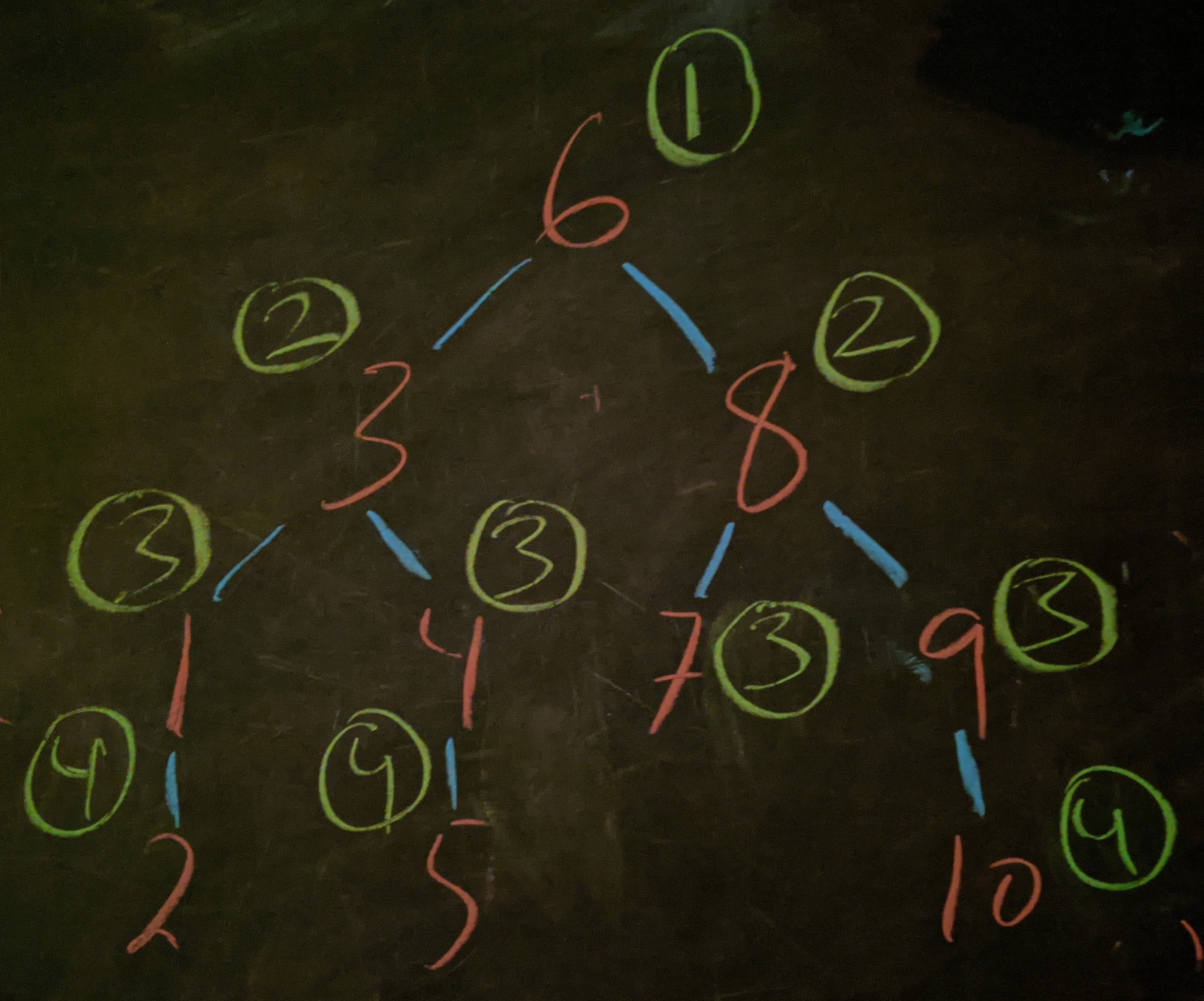Question: Every night your children change their favorite word and refuse to let you go to bed until you guess which word it is. If you guess wrong, they’ll tell you if their word comes before or after yours in a list of all words. If there are $W = 267,751$ words that they’re knowledgable about, how many guesses will you take, on average, to uncover their word?

## Solution

First of all, it doesn’t matter whether these are words, or pictures, or numbers — so long as they can be ordered it’s the same problem.

### Small cases

Let’s think about how things should go if we had $3$ items. If we first asked if the number was $1$ that would be a waste, because in $2/3$ of the cases, it wouldn’t be, and we’d have to ask a second question, and then a third, for an average of $2$ questions. By contrast, if we asked about $2$ first, we would have to ask at most $1$ more question, resulting in an average of $5/3 \approx 1.67$ questions.

Similarly, if we had $7$ items, we could ask about $4$ first. $1/7$ of the time we’ll learn that we guessed right while $3/7$ of the time we’ll have guessed too high and the other $3/7$ of the time we’ll have guessed too low. If we guessed too high or too low, then we’re back in the $3$-number case. So, on average, we’d need

$1\times\frac17 + 2\times\frac27 + (2 + 1)\times\frac47 = 17/7 \approx 2.43$

The pattern here is that in every layer we double the number of numbers, and that each node is halfway between the nodes that emerge from it. These are called binary trees.

For trees like this, the number of questions we need to ask is one less than the number of layers. We can make trees like this of size $1, 1+2=3,1+2+4=7,1+2+4+8=15, \ldots$ and so on. The $n^\text{th}$ layer in such a tree contains $2^{n-1}$ numbers.

### Questions for a binary tree

If the tree has $n$ layers, then it has a total of

$2^0 + 2^1 + 2^2 + \cdots + 2^{n-1} + 2^n$

nodes in it, which comes out to $2^{n+1} - 1.$

If the kids pick their word at random, then there will be $1$ node that takes $1$ question, $2$ nodes that take $2$ questions, $4$ nodes that take $3$ questions, and so on, leading to

$\langle Q\rangle = \dfrac{\sum\limits^n i \times 2^{i-1}}{\sum\limits^n 2^{i-1}} = \dfrac{2^n(n-1)+1}{2^n-1}$

Plugging in $n=2$ and $n=3$ as a sanity check, we get $5/3$ and $17/7,$ as expected.

### Non-binary trees

For dictionaries with $2^n - 1$ words, the question format implicates the $n$-layer binary tree as the natural strategy for extracting the secret word. It’s as efficient as possible given the means of interrogation.

That’s great, but $W = 267,751$ is not of the form $2^n - 1.$ What do we do when the number of words is not equal to $1$ less than a power of $2$?

Well, $L = \lfloor\log_2 W \rfloor = 18,$ so $267,751$ is $(2^{18} - 1)$ with $5608$ left over.

Perhaps we could put $(2^{18}-1)$ of the numbers into an $18$-layer binary tree, and put the first $5608$ numbers into their own set. Surely, we could sift through the $5608$ numbers in fewer questions than the smallest binary tree that could contain them, which can hold $2^{13} - 1$ numbers.

However, this gives a guaranteed extra question for all $267,751$ numbers (to tell which tree it’s in) in exchange for getting just under $(2^{13}\times 12 + 1)/(2^{13}-1) \approx 12$ questions on $5608$ of the numbers.

That doesn’t seem worth it.

### Decorated binary trees

In fact, the best strategy is still to use a single binary tree.

We simply put $2^{18}-1$ of the numbers into a binary tree and hang the $5608$ leftover words off the bottom like ornaments. Each of the extra nodes requires $(18+1)$ questions reflecting the $18$ needed to get to the end of the binary tree plus $1$ extra question to distinguish it from the number it hangs from.

For example, a $(W,L) = (10,3)$ tree is laid out below.Proof of concept for laying out a tree of $W=10$ words, with $L=\lfloor\log_2 W\rfloor = 3$ layers in the core binary tree. The numbers are shown in red while the number of questions they require is shown in green. By inspection, $\langle Q\rangle = 29/10,$ in agreement with the prediction of the formula.

This brings the average number of questions to

$\langle Q\rangle = \dfrac{2^L(L-1) + 1 + (L+1)(W - (2^L-1))}{W}$

where $L = \lfloor\log_2 W\rfloor,$ as before.

Plugging in $(W,L) = (267751, 18),$ we get

$\langle Q\rangle = \dfrac{4,563,001}{267,751} \approx 17.042\,\text{questions}$